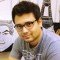Home » Core Java » text » DecimalFormat » Java Decimal Format ExampleAnirudh is a Java programmer with extensive experience in building Java/J2EE applications. He has always been fascinated by the new technologies and emerging trends in software development. He has been involved in propagating these changes and new technologies in his projects. He is an avid blogger and agile enthusiast who believes in writing clean and well tested code.

# Java Decimal Format Example

In this example we will see how we can use the `DecimalFormat`class to format decimal numbers. This class is useful when we want to format the decimal numbers in following ways :

• As per a defined specific string pattern

We can specify a pattern string to define the display of the decimal.

• Specific to a locale

We can use locale-specific settings to control the application of pattern for display of decimals.

Lets see each of the cases in the examples one by one:

### 1) Using a format pattern string

```package com.javacodegeeks.example;

import java.text.DecimalFormat;

private static final String COMMA_SEPERATED = "###,###.###";
private static double number = 12345.6;

public static void main(String[] args) {

DecimalFormat decimalFormat = new DecimalFormat(COMMA_SEPERATED);
System.out.println(decimalFormat.format(number));
}
}
```

Output :

```12,345.6
```

In the above example we have defined a String PATERN which is passed as a parameter to the constructor of class `DecimalFormat`. Then we used the method `format`, in which we passed the decimal and it returned the desired formatted decimal string.

We can change this pattern later by using the method `applyPattern()`.
Lets suppose we want show the number with two decimal places. Lets see an example of how to do it:

```...
private static final String TWO_DECIMAL_PLACES_WITH_COMMA = "###,###.00";
System.out.println("After another pattern");
decimalFormat.applyPattern(TWO_DECIMAL_PLACES_WITH_COMMA);
System.out.println(decimalFormat.format(number));
```

Output:

```After another pattern
12,345.60
```

So, different patterns can be used to achieve different patterns, below are few examples :

```package com.javacodegeeks.example;

import java.text.DecimalFormat;

private static final String FIXED_PLACES = "000,000.00";
private static final String BEGIN_WITH_DOLLAR = "\$###,###.00";

private static double number = 12345.6;

public static void main(String[] args) {

applyCustomFormat(BEGIN_WITH_DOLLAR, number);
applyCustomFormat(FIXED_PLACES, number);
}

private static void applyCustomFormat(String pattern, double value) {
DecimalFormat decimalFormat = new DecimalFormat(pattern);
decimalFormat.applyPattern(pattern);
System.out.println(decimalFormat.format(value));
}
}
```

Output:

```\$12,345.60
012,345.60
```

### 2) Using locale-specific pattern

In the previous examples we created a DecimalFormat for the default Locale of the JVM, where the code is running. In case we want to display the numbers as per the locale, we would create an object of the class `NumberFormat` passing it the locale and then cast it to `DecimalFormat`.

Lets see an example :

```.....
Locale UK_LOCALE = new Locale("en", "UK");
Locale US_LOCALE = new Locale("en","US");
applyCustomFormat(COMMA_SEPERATED, number, UK_LOCALE);
applyCustomFormat(BEGIN_WITH_DOLLAR, number, US_LOCALE);

```
```private static void applyCustomFormat(String pattern, double value, Locale locale) {
DecimalFormat decimalFormat = (DecimalFormat) NumberFormat.getNumberInstance(locale);
System.out.println(decimalFormat.format(value));

}
```

Output:

```12,345.6
12,345.6
```

Here, the system locale is set to UK so the output is been formatted as per the UK locale.

There are few other methods and scenarios which can be useful, lets see them :

#### Grouping

We can group the digits using the method `setGroupingSize(integer`). This method groups the numbers starting from the decimal.

Lets see an example :

```                 ...
//setting group
DecimalFormat groupeddecimalFormat = new DecimalFormat("###,###.###");
groupeddecimalFormat.setGroupingSize(4);
System.out.println(groupeddecimalFormat.format(13243534.32));
....
```

Output :

```13,243,534.32
```

In the above example we can see that the large number is grouped into 4 integers, also observe that the pattern applied is overwritten by the group set method.

# Do you want to know how to develop your skillset to become a Java Rockstar?

## Subscribe to our newsletter to start Rocking right now!

### and many more ....